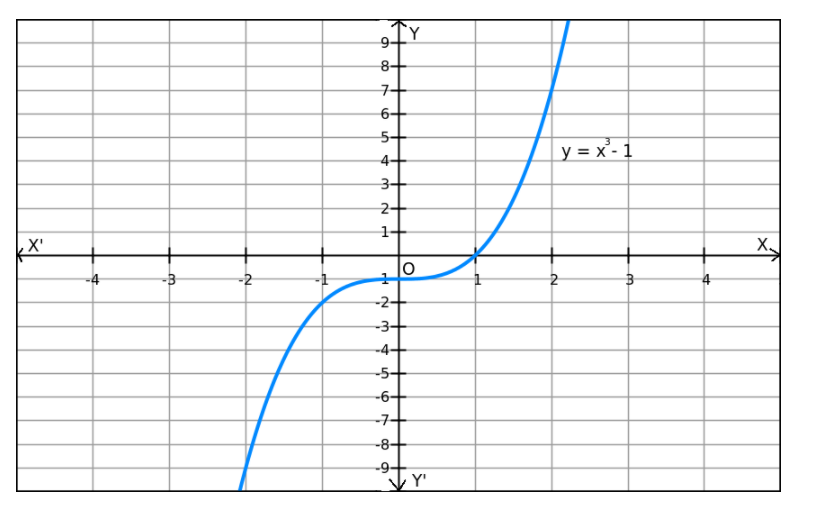Deepak Scored 45->99%ile with Bounce Back Crack Course. You can do it too!

# If the solve the problem

Question:

$f(x)=x^{3}-1$ on $R$

Solution:We can observe that f(x) increases when the values of x increase and f(x) decreases when the values of x decrease. Also, f(x) can be reduced by giving smaller values of x.
Similarly, f(x) can be enlarged by giving larger values of x.
So, f(x) does not have a minimum or maximum value.